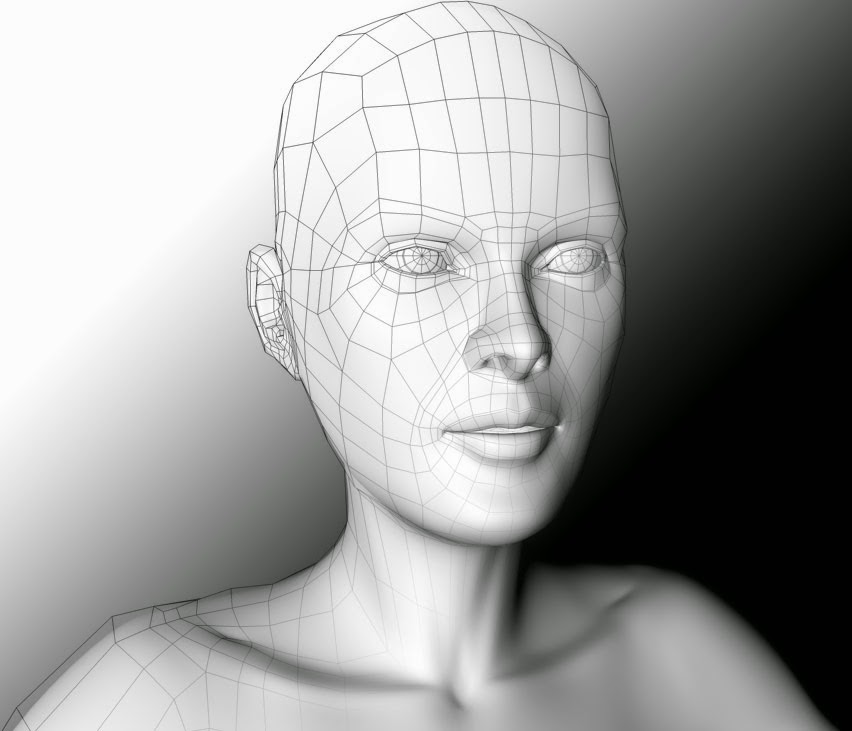3D Modelling Software Screenshot

This article on 3D modelling is the first of 5 articles on the topic of 3D Graphics and Animation. In this instance, 3D graphics means computer generated three-dimensional graphics. In 3D graphics, elements are visualized by creating three-dimensional models out of them. The final image is rendered from a virtual scene that utilizes several elements which are familiar from real life such as lights, materials and cameras. 3D graphics can be seen in multiple different forms such as an image, an animation or real time visualization inside of a computer game.

The creation of 3D graphics is a complicated process and therefore is, in my opinion, easier to understand when discussed in small pieces, hence the 5 article series. First up it's 3D Modelling which refers to the creation of the 3D model itself.

## 3D Modelling - The Parts...

A 3D model is a mathematical representation of an object. 3D modelling can be compared to sculpting. Artist builds or moulds a 3D object by taking into account all different sides and angles. 3D models consist of smaller elements (vertex, edge, face, polygon) which can be manipulated individually in whatever software you are using to model your 3D object, such as Blender, Maya or 3D Studio Max.

### Vertex: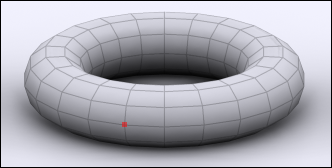vertex

Vertex is the smallest building block of a 3D model. Vertex is a point where two or more edges meet and where corners of faces or polygons meet. In a 3D model a vertex is shared between all connecting edges, faces and polygons. Transforming a vertex affects all connected edges, faces and polygons.

### Edge: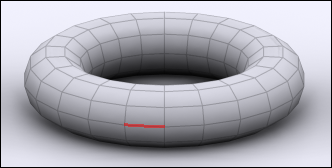edge

Edge is a line between two vertices. Edges are border lines of faces and polygons. In a 3D model an edge is shared between two adjacent faces or polygons. Transforming an edge affects all connected vertices, faces and polygons.

### Face: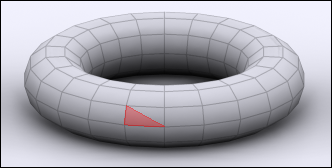face

Face is a triangle. Face is a surface between three corner vertices and three surrounding edges. Transforming a face affects all connected vertices, edges and polygons.

### Polygon:polygon

Polygon is an even surface which has four or more corners and is made of two or more faces. A polygon is surrounded by edges and has a vertex in each corner. Animated high quality 3D characters are often made mostly of four-sided polygons. Polygons with 5 or more sides can cause problems in deforming surfaces such as a human face.

## 3D Modelling - The Techniques...

3D models can be created in many different ways. The choice of modelling technique depends on the requirements and the complexity of the object. The following list describes some of popular 3D modelling techniques:

### Standard Primitives and Modifiers:Primitives and Modifiers

Many 3D software packages include tools for creating standard objects such as boxes and spheres easily. One of the simplest 3D modelling techniques is to combine these standard objects to create complex 3D models. 3D Studio MAX includes standard objects such as sphere, cube and tube. These standard objects can be modified through their parameters (radius, height etc.) or through special modifiers (stretch, bend etc.). By combining several different standard objects and by modifying them, one can create complex 3D models.

### Polygon Modelling: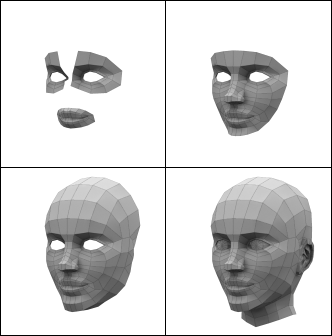Stages of Polygon Modelling

Complex objects are often modeled polygon by polygon. 3D software packages include many efficient tools for creating and manipulating polygons.
Subdivision surface means a surface which is created by dividing the original 3D model into smaller polygons. At the same time 3D model's corners become rounder and the surface becomes smoother. Subdivision surfaces is a very popular modelling technique. The advantage of a subdivision surface is the fact that one can create a coarse 3D model which is then automatically subdivided into a smoother surface.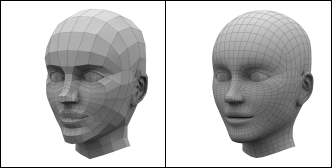A Polygon Model Before and After Subdivision

### Boolean Operations:

The starting point of Boolean operation is two overlapping 3D objects. Boolean operations are prone to error and the resulting geometry might have underlying problems.Boolean Modelling

#### Boolean operation has four possible results:The 4 Possible Outcomes of a Boolean Operation
• Union: Two 3D models are combined and the unnecessary geometry inside of the Models is removed.
• Intersection: Overlapping a part of the two 3D objects.
• Subtraction (A-B): Object A is subtracted from object B.
• Subtraction (B-A): Object B is subtracted from object A.

### NURBS:

NURBS stands for Non-Uniform Rational B-spline. In NURBS modelling, lines and surfaces are not manipulated by moving vertices, edges, faces or polygons. Instead NURBS surfaces and lines are manipulated by special control points. The following 2 techniques make use of NURBS.

### Solid of Revolution:A cross section and the object created by a solid of revolution

Solid of revolution is made of one line. The line represents a half cross-section of the object. Solid of revolution is created by revolving the line around a specified axis which then creates the form of the object in 3D.

### Lines:A 3D object and the cage of lines used to create it

3D objects can be created by creating a cage of lines which can be then be converted to a solid 3D surface. This can be a difficult technique to master as it can be hard to visualise the outcome of the lines once they are solidified but once mastered it allows for very quick modelling of objects.

Now that you understand the basics of 3D modelling, why not give it a try, it won't cost you a penny either! We recommend the free software Blender to get you started but there are many other great free 3D modellling softwares. The tutorials linked to below are also a good place to learn the basics of modelling 3D objects.

Or, if you're not ready for that yet, read the next tutorial in the 3D Modelling and Animation series - 3D Lighting techniques.

3D Modelling Basics & Terminology3DReviewed by Opus Web Design on July 13, 2016 Rating: 5